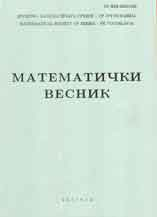eLibrary of Mathematical Instituteof the Serbian Academy of Sciences and Arts> Home / All Journals / Journal /Matematički VesnikPublisher: Društvo matematičara Srbije, BeogradISSN: 0025-5165 (Print), 2406-0682 (Online)Issue: 66_2Date: 2014Journal Homepage

 On $(f,g)$-derivations of $B$-algebras 125 - 132 L. Kamali Ardekani and B. Davvaz
AbstractKeywords: Commutative $B$-algebra, $f$-derivation, $(f,g)$-derivation.MSC: 06F35 16B70 16W25
 Generalizations of primal ideals over commutative semirings 133 - 139 Malik Bataineh and Ruba Malas
AbstractKeywords: Primal ideal, $\phi$-prime ideal, weakly primal ideal, $\phi$-primal ideal.MSC: 13A15 16Y60
 Property ($gR$) under nilpotent commuting perturbation 140 - 147 O. García, C. Carpintero, E. Rosas and J. Sanabria
AbstractKeywords: Property ($gR$), semi B-Fredholm operator, perturbation.MSC: 47A10 47A11 47A53 47A55
 On an inequality of Paul Turán 148 - 154 B. A. Zargar
AbstractKeywords: Inequalities, zeros of polynomials, derivative, maximum modulus.MSC: 30C10 30C15
 Approximation of functions belonging to the generalized Lipschitz class by $C^{1}\cdot N_{p}$ summability method of conjugate series of Fourier series 155 - 164 Vishnu Narayan Mishra, Kejal Khatri and Lakshmi Narayan Mishra
AbstractKeywords: Generalized Lipschitz $W(L_r,\xi(t))$-class of functions, conjugate Fourier series, degree of approximation, $C^1$ means, $N_p$ means, product summability $C^{1}\cdot N_{p}$ transform.MSC: 40G05 42B05 42B08
 On $\Cal{I}$ and $\Cal{I}^*$-equal convergence and an Egoroff-type theorem 165 - 177 Pratulananda Das, Sudipta Dutta and Sudip Kumar Pal
AbstractKeywords: Ideal, filter, $\Cal{I}$ and $\Cal{I}^*$-equal convergence, $P$-ideal, Chain condition, $\Cal{I}^*$-uniform equal convergence, $\Cal{I}^*$-almost uniform equal convergence, $\Cal{I}^*$-quasi vanishing restriction, Egoroff Theorem.MSC: 40G15 40A99 46A99
 Set-valued Prešić-Ćirić type contraction in 0-complete partial metric spaces 178 - 189 Satish Shukla
AbstractKeywords: Set-valued mapping, partial metric space, fixed point, Prešić type mapping.MSC: 47H10 54H25
 Coupled fixed point theorems in $G_{b}$-metric spaces 190 - 201 Shaban Sedghi, Nabi Shobkolaei, Jamal Rezaei Roshan and Wasfi Shatanawi
AbstractKeywords: Common fixed point, coupled coincidence fixed point, $b$-metric space, $G$-metric space, generalized $b$-metric space.MSC: 54H25 47H10 54E50
 A companion of Grüss type inequality for Riemann-Stieltjes integral and applications 202 - 212 Mohammad W. Alomari
AbstractKeywords: Ostrowski's inequality, bounded variation, Riemann-Stieltjes integral.MSC: 26D15 26D20 41A55
 Growth and oscillation of meromorphic solutions of linear difference equations 213 - 222 Zinelaâbidine Latreuch and Benharrat Belaïdi
AbstractKeywords: Linear difference equations, meromorphic function, order of growth, type of meromorphic function.MSC: 39A10 30D35 39A12
Article page: 12>>

 Remote Address: 44.200.175.255 • Server: elib.mi.sanu.ac.rsHTTP User Agent: CCBot/2.0 (https://commoncrawl.org/faq/)